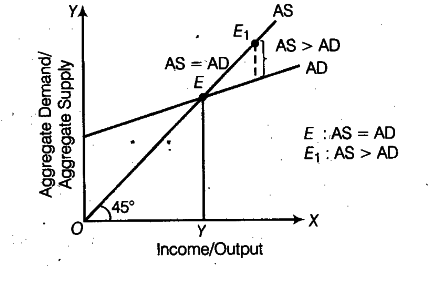# In an economy Aggregate Demand

In an economy Aggregate Demand is less than Aggregate Supply. Is the economy in equilibrium? If not, explain the changes that will bring the economy in equilibrium.

If Aggregate Demand (AD) is less than Aggregate Supply (AS), then economy is not in equilibrium because an economy is in equilibrium when AS = AD. When AD < AS, flow of goods and services in the economy tends to exceed then demand. As a result, some of the goods would remain unsold. To clear unwanted stocks, the producers would plan a cut in production. Consequently, AS will reduce to become equal to AD. This is how AS adapts itself to AD.
In the following figure, equilibrium is struck at point E where AD = AS. At point {{E}_{1}} Aggregate Supply exceeds Aggregate Demand.It will cause following changes in the economy:
(i) Stock of the producers would be in excess of the desired limit.
(n) Profits will start shrinking.
(iii) Desired level of output for the subsequent year will face a cut.
(iv) Levels of income and employment will tend to shrink to the point, where S = I, corresponding
to point E in the diagram. Thus, the economy will come back to the state of equilibrium